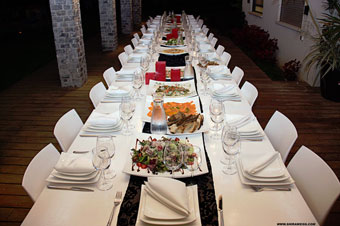Critical Section

 eight people to dinner, solved Wednesday,  02/11/15  08:46 PM

The other day I asked:

Eight people are seated at a circular table. Each person gets up and sits down again_either in the same chair or in the chair immediately to the left or right of the one they were in. How many different ways can the eight people be reseated?

So ... I know you've all been working on the problem ... want to know the answer?  No, it's not 42, it's ... 49!  Is that what you got?Here's how I got it.  Basically each person can either stay where they are or swap with the person next to them.  If they don't stay where they are and don't swap, then you end up with an unused chair and someone who has no chair, and that's not allowed.

Let's first consider a table which is NOT round.  Let's call N(x) the number of ways people can be reseated at such a table, where x is the total number of people and seats.

The first person can either stay where they are, or they can swap with their only neighbor.  If they stay where they are, there are N(x-1) remaining possibilities.  If they swap, there are N(x-2) remaining possibilities.  That means N(x) = N(x-1) + N(x-2). This happens to be the Fibonacci sequence.  Who knew Fibonacci was a waiter?

Next lets consider a table which IS round.  Let's call R(x) the number of ways people can be reseated at such a table, where x is the total number of people and seats.

The first person either stays where they are, or they can swap with their left neighbor, or swap with their right neighbor.  If they stay where they are, there are N(x-1) remaining possibilities.  (See what I did there?*)  If they swap, there are N(x-2) remaining possibilities*.  They have two neighbors with whom they can swap.  So R(x) = N(x-1) + 2N(x-2).  Except ... with a round table there are two other possibilities, everyone can swap right, and everyone can swap left.  So R(x) = N(x-1) + 2N(x-2) + 2.

Okay... now we can solve the problem:

N(1) = 1
N(2) = 2
N(3) = 1+2 = 3
N(4) = 2+3 = 5
N(5) = 3+5 = 8
N(6) = 5+8 = 13
N(7) = 8+13 = 21

R(8) = N(7) + 2N(6) + 2 = 21 + 2(13) + 2 = 49

Bon appetit!

* after the first person decides, the rest of the round table becomes "straight"

Greatest Hits
Correlation vs. Causality
The Tyranny of Email
Unnatural Selection
Lying
Aperio's Mission = Automating Pathology
On Blame
Try, or Try Not
Books and Wine
Emergent Properties
God and Beauty
Moving Mount Fuji The Nest Rock 'n Roll
IQ and Populations
Are You a Bright?
Confidence
The Joy of Craftsmanship
The Emperor's New Code
Toy Story
The Return of the King
Religion vs IQ
In the Wet
the big day
solving bongard problems
visiting Titan
unintelligent design
the nuclear option
estimating in meatspace
second gear
On the Persistence of Bad Design...
Texas chili cookoff
almost famous design and stochastic debugging
may I take your order?
universal healthcare
entertainment
triple double
New Yorker covers
Death Rider! (da da dum)
how did I get here (Mt.Whitney)?
the Law of Significance
Holiday Inn
Daniel Jacoby's photographs
the first bird
Gödel Escher Bach: Birthday Cantatatata
Father's Day (in pictures)
your cat for my car
Jobsnotes of note
world population map
no joy in Baker
vote smart
exact nonsense
introducing eyesFinder
resolved
to space
notebooks
where are the desktop apps?